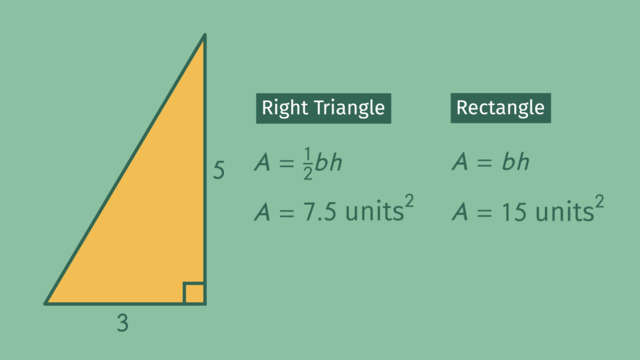# Finding the Area of a Right TriangleRating

Be the first to give a rating!

The authorsChris S.

## Basics on the topicFinding the Area of a Right Triangle

After this lesson you will be able to justify the area formula for a right triangle.

The lesson begins with defining the area of a rectangle and drawing a diagonal to create two identical right triangles. It leads to discovering that every right triangle is one half the area of a rectangle. It concludes with using the area of a triangle formula to calculate area.

Learn how to find the area of right triangles by helping Mofa pitch his project to the Mayor to make their city a better place!

This video includes key concepts, notation, and vocabulary such as: right triangle (a triangle containing a 90° interior angle); area of a rectangle (area equals base times height); and area of a triangle (area equals one half base times height).

Before watching this video, you should already be familiar with finding the area of a rectangle or square, and that shapes can be composed of other shapes.

After watching this video, you will be prepared to learn more about right triangles including the relationship between the length of the hypotenuse and the other sides.

Common Core Standard(s) in focus: 6.G.A.1 A video intended for math students in the 6th grade Recommended for students who are 11-12 years old

### TranscriptFinding the Area of a Right Triangle

The notorious biker, Mofa, has two passions in life: biking and volunteer work? This tough guy-slash-responsible citizen is pitching a project to the Mayor which he hopes will make their city a better place to live. Mofa proposes to transform some unused triangular corners in the city into public vegetable gardens! But the mayor is no pushover. To present a convincing proposal Mofa will need to find the area of right triangles. The gardens Mofa wants to build will all be shaped like right triangles, so they can fit easily into unused nooks and crannies around the city. We know this garden is a right triangle, because two of its sides share a right angle. As part of his proposal, Mofa needs to figure out the area of each garden, so he can calculate amounts of soil, fertilizer, and seeds. To understand the area of a right triangle, let's first look at the area of a rectangle. The area of any rectangle is base times height. This rectangle has a base of 3 and a height of 5. Plugging those values into our formula we see it has an area of 15 square units. If we draw a diagonal here that results in two right triangles. How do you think the areas of these two triangles relate to each other? Is one bigger than the other? Or are they the same? If we place one triangle on top of the other we can see that these two triangles are, indeed, the same size. So, how would you calculate the area of one of these right triangles? If the area of a rectangle is base times height that would make the area of one of our triangles, half of that. That's half of 3 times 5 which is 7.5 square units. Now let's take this formula with us and help Mofa calculate the area of his proposed gardens. Here's one of the vacant lots that Mofa wants to convert to a green oasis in the city. To calculate the area of this garden, we need to identify the base and the height. Which number should we use for our base? Usually we call the bottom side our base so let's plug 8 into the formula for 'b'. That makes 15 our height, 'h', because that side is perpendicular to the base. Multiplying one half times 8 gives us 4 and 4 times 15 gives us 60 square yards. Let's check our work, but just to make it interesting what if we call this side the base? And this side is the height? Will the area be the same? Substituting 15 in for our base and 8 for our height. We see that our area is still 60 square yards. The mayor is definitely going to be impressed. Let's take a look at another garden that Mofa has planned. Can you identify the base and height? Normally we call the bottom side of a triangle the base, but this doesn't have a "bottom" so let's rotate it slightly, since we know that won't change the area. Now, can we use one of the other two sides for our height? Remember the base and height must be perpendicular to each other, so neither of these sides will work. But with the triangle in this position we have a base and height which are perpendicular. Now we can just plug in 21.5 for 'b' in our formula and 17.2 for 'h'. Multiplying gives us an area of 184.9 square yards. It's a sizeable garden, but the Mayor has got to respect Mofa's commitment to building a greener city! While she's considering his proposal let's review how to find the area of a right triangle. Every right triangle can be visualized as one half of a rectangle. That's why we can calculate their area by multiplying one-half the base times the height. To find the base and height, just look for the sides that are perpendicular to each other. After a few months of hard work, Mofa's gardens are ready for harvest! So he invites some of his biker friends to enjoy the spoils of his labor. But maybe motorcycles and urban gardening aren't always a good combination.